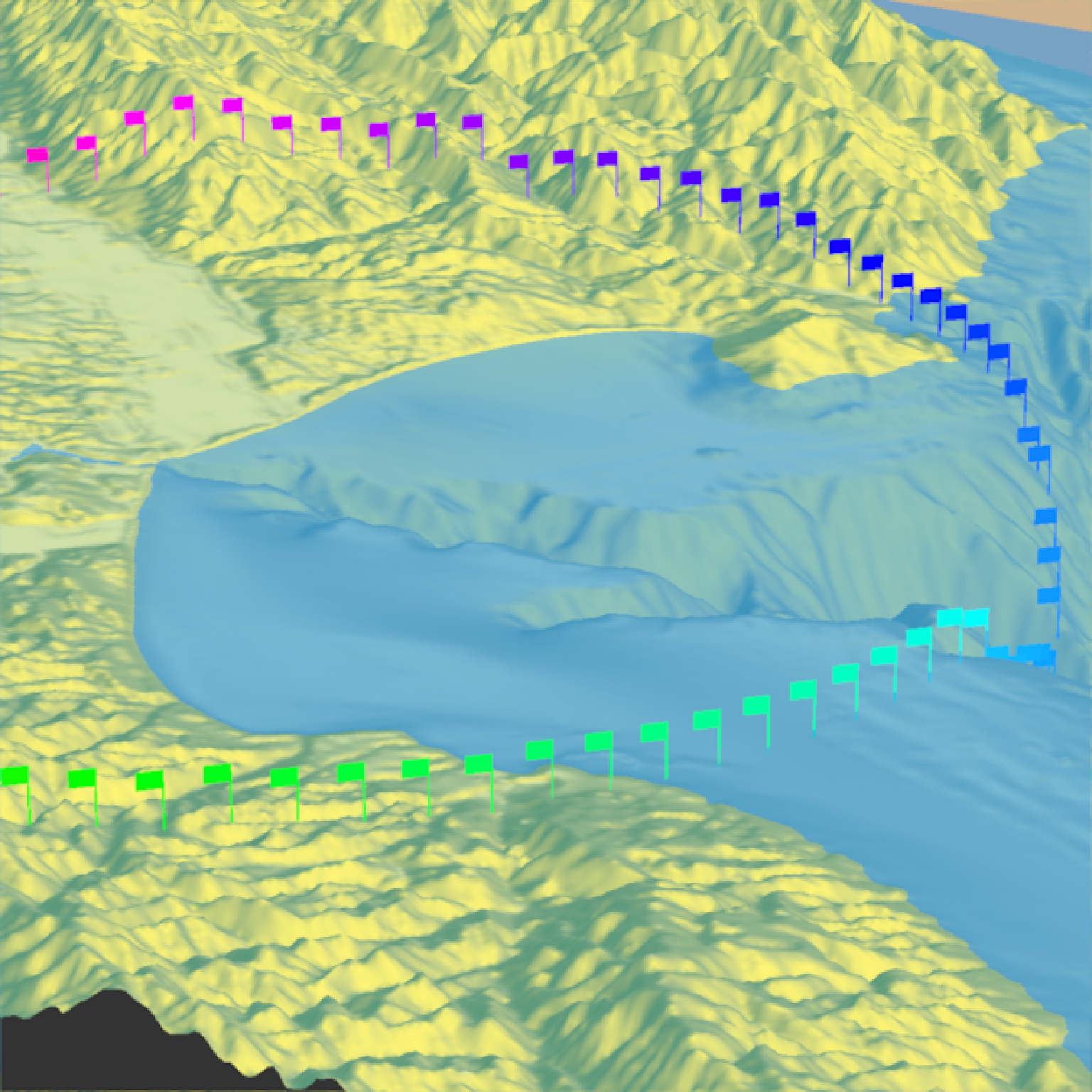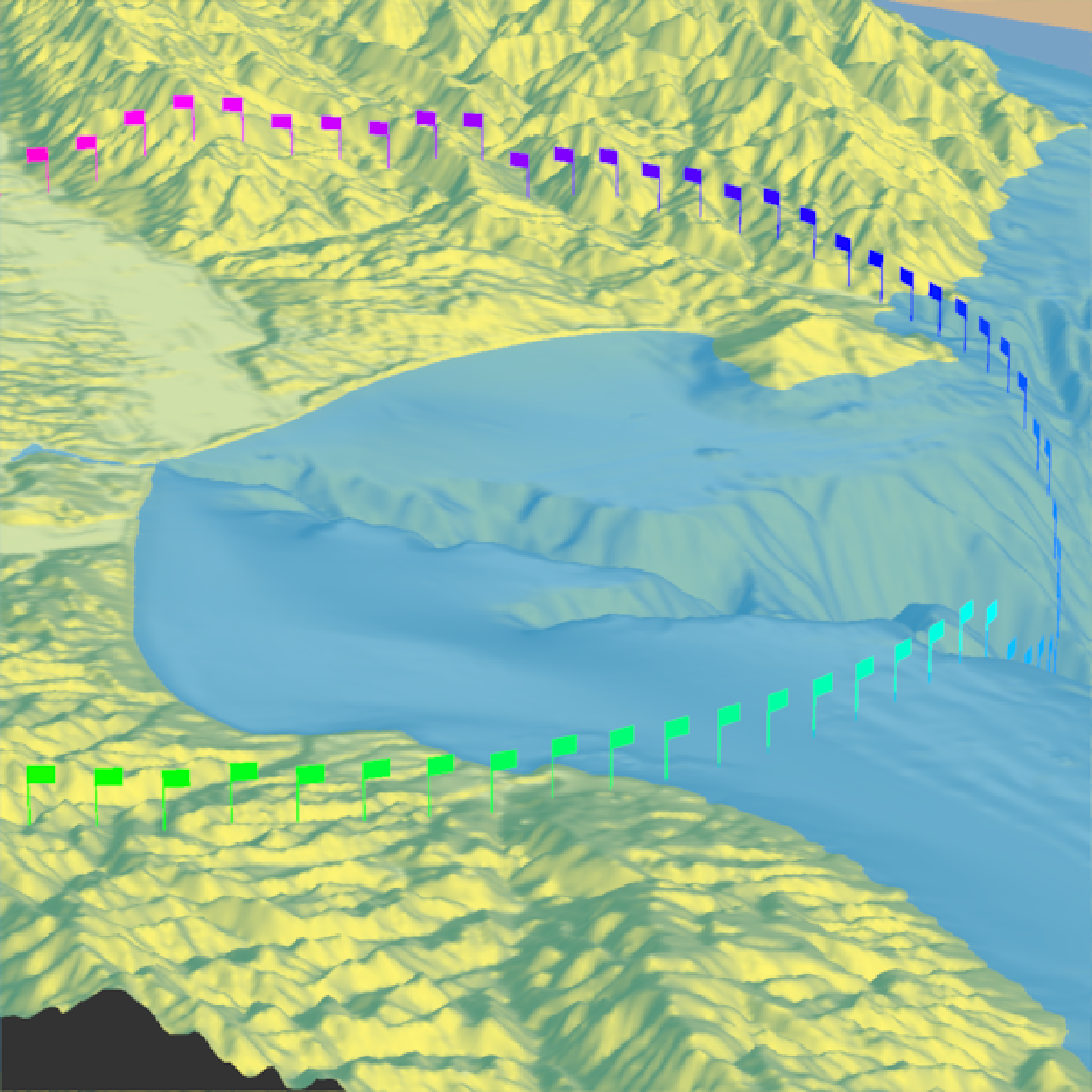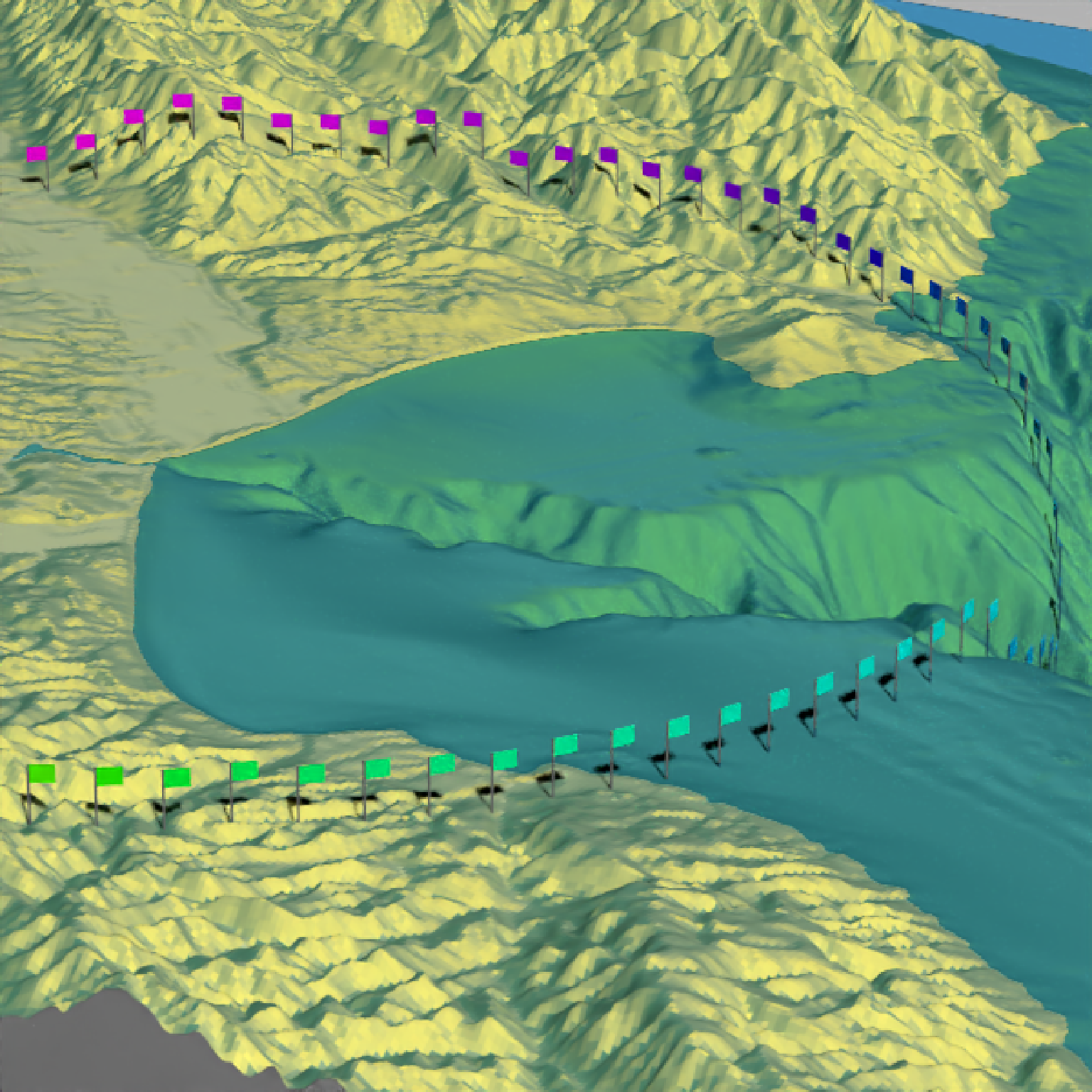Adds 3D OBJ model to the current scene, using latitude/longitude or coordinates in the reference system defined by the extent object. If no altitude is provided, the OBJ will be elevated a constant offset above the heightmap. If the OBJ goes off the edge, the OBJ will be filtered out.

If no latitudes or longitudes are passed in, the OBJ will be plotted in the coordinate system set by the user-specified extent argument as-is. Use this alongside save_multipolygonz_to_obj() to plot 3D polygons imported from geospatial sources in the proper location (but for ease of use, use render_multipolygonz() to plot this data directly).

render_obj(
filename,
extent = NULL,
lat = NULL,
long = NULL,
altitude = NULL,
xyz = NULL,
zscale = 1,
heightmap = NULL,
color = "grey50",
offset = 0,
obj_zscale = FALSE,
swap_yz = NULL,
angle = c(0, 0, 0),
scale = c(1, 1, 1),
clear_previous = FALSE,
baseshape = "rectangle",
rgl_tag = "",
...
)

## Arguments

filename

Filename for the OBJ file.

extent

Either an object representing the spatial extent of the scene (either from the raster, terra, sf, or sp packages), a length-4 numeric vector specifying c("xmin", "xmax","ymin","ymax"), or the spatial object (from the previously aforementioned packages) which will be automatically converted to an extent object.

lat

Vector of latitudes (or other coordinate in the same coordinate reference system as extent).

long

Vector of longitudes (or other coordinate in the same coordinate reference system as extent).

altitude

Default NULL. Elevation of each point, in units of the elevation matrix (scaled by zscale). If left NULL, this will be just the elevation value at ths surface, offset by offset. If a single value, the OBJ will be rendered at that altitude.

xyz

Default NULL, ignored. A 3 column numeric matrix, with each row specifying the x/y/z coordinates of the OBJ model(s). Overrides lat/long/altitude and ignores extent to plot the OBJ in raw rgl coordinates.

zscale

Default 1. The ratio between the x and y spacing (which are assumed to be equal) and the z axis in the original heightmap.

heightmap

Default NULL. Automatically extracted from the rgl window--only use if auto-extraction of matrix extent isn't working. A two-dimensional matrix, where each entry in the matrix is the elevation at that point. All points are assumed to be evenly spaced.

Default TRUE. Whether to load the accompanying MTL file to load materials for the 3D model.

Default TRUE. Whether to load normals for the 3D model.

color

Default black. Color of the 3D model, if load_material = FALSE.

offset

Default 5. Offset of the track from the surface, if altitude = NULL.

obj_zscale

Default FALSE. Whether to scale the size of the OBJ by zscale to have it match the size of the map. If zscale is very big, this will make the model very small.

swap_yz

Default NULL, defaults to FALSE unless plotting raw coordinates (no lat or long passed). Whether to swap and Y and Z axes. (Y axis is vertical in rayshader coordinates, but data is often provided with Z being vertical).

angle

Default c(0,0,0). Angle of rotation around the x, y, and z axes. If this is a matrix or list, each row (or list entry) specifies the rotation of the nth model specified (number of rows/length of list must equal the length of lat/long).

scale

Default c(1,1,1). Amount to scale the 3D model in the x, y, and z axes. If this is a matrix or list, each row (or list entry) specifies the scale of the nth model specified (number of rows/length of list must equal the length of lat/long).

clear_previous

Default FALSE. If TRUE, it will clear all existing points.

baseshape

Default rectangle. Shape of the base. Options are c("rectangle","circle","hex").

rgl_tag

Default "". Tag to add to the rgl scene id, will be prefixed by "obj"

...

Additional arguments to pass to rgl::triangles3d().

## Examples

if(rayshader:::run_documentation()) {
#Render the 3D map
moss_landing_coord = c(36.806807, -121.793332)
montereybay %>%
plot_3d(montereybay,zscale=50,water=TRUE,
theta=210,  phi=22, zoom=0.20, fov=55)

t = seq(0,2*pi,length.out=100)
circle_coords_lat = moss_landing_coord + 0.3 * sin(t)
circle_coords_long = moss_landing_coord + 0.3 * cos(t)

#Create a rainbow spectrum of flags
render_obj(flag_full_obj(), extent = attr(montereybay,"extent"), heightmap = montereybay,
lat = unlist(circle_coords_lat), long = unlist(circle_coords_long),
scale=c(2,2,2), angle=c(0,45,0),
zscale=50, color=rainbow(100), smooth = FALSE, clear_previous = TRUE)
render_snapshot()
}#Rotate the flag to follow the circle
render_obj(flag_full_obj(), extent = attr(montereybay,"extent"), heightmap = montereybay,
lat = unlist(circle_coords_lat), long = unlist(circle_coords_long),
scale=c(2,2,2),
angle=matrix(c(rep(0,100), seq(0,-360,length.out=101)[-1],rep(0,100)),ncol=3),
zscale=50, color=rainbow(100), smooth = FALSE, clear_previous = TRUE)
render_snapshot()
}#Style the pole with a different color
render_obj(flag_pole_obj(), extent = attr(montereybay,"extent"), heightmap = montereybay,
lat = unlist(circle_coords_lat), long = unlist(circle_coords_long),
scale=c(2,2,2),
angle=matrix(c(rep(0,100), seq(0,-360,length.out=101)[-1],rep(0,100)),ncol=3),
zscale=50, color="grey20", smooth = FALSE, clear_previous = TRUE)
render_obj(flag_banner_obj(), extent = attr(montereybay,"extent"), heightmap = montereybay,
lat = unlist(circle_coords_lat), long = unlist(circle_coords_long),
scale=c(2,2,2),
angle=matrix(c(rep(0,100), seq(0,-360,length.out=101)[-1],rep(0,100)),ncol=3),
zscale=50, color=rainbow(100), smooth = FALSE)

#And all of these work with render_highquality()
render_highquality(sample_method="sobol_blue",clamp_value=10)
}